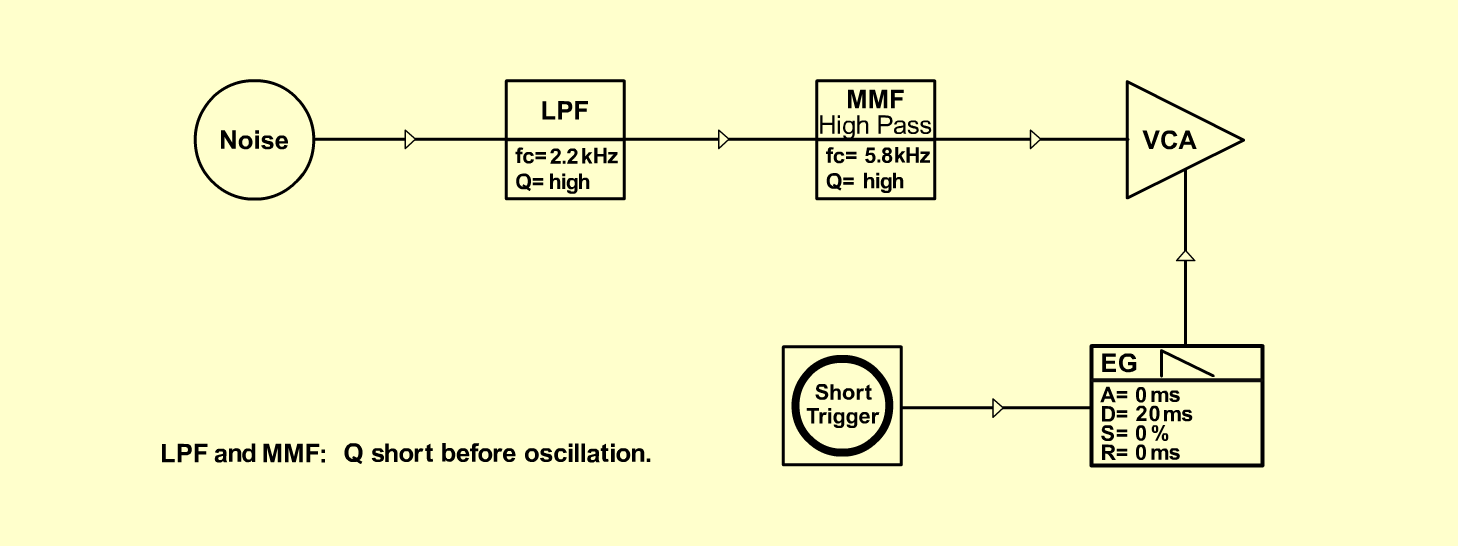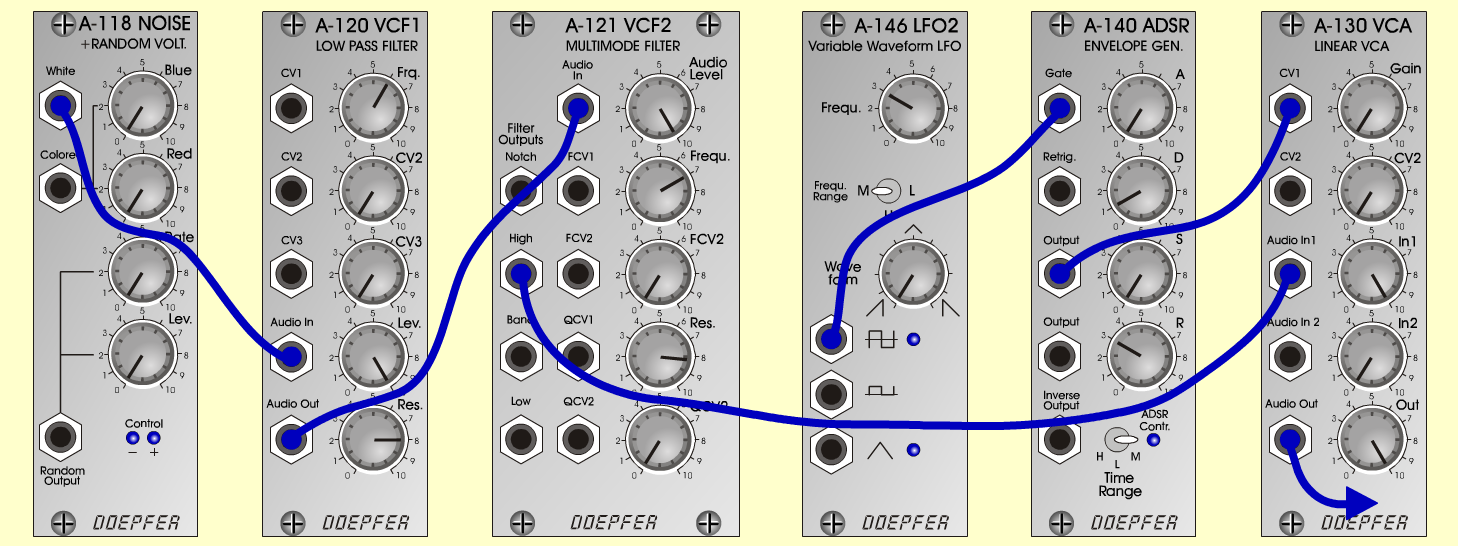ClavesD r u m sD o e p f e r A - 100 Connections: Settings: A-118 (White)  <=>   A-120 (Audio In)   A-120 (Out) <=>   A-121 (Audio In)   A-121 (High) <=>   A-131 (Audio In 1)   A short trigger: KB, Seq or   A-146 (Square) <=>   A-140 (Gate)   A-140 (Output) <=>   A-131 (CV 1) A-120 (Freq = 6, Lev = 10, Res = 8)   A-121 (Audio Level = 10, Freq = 7, Res = 8.2)   A-146 (Freq = 3, Wave Form = 0, Range = M)   A-140 (A = 0, D = 1, S = 0, R = 3, Range = M)   A-131 (Gain = 0, Audio In 1 = 10, Audio Out = 10) Notes: Set Q from A-120 and A-121 short before   Oscillation.   Pitch: Change A-120 (Freq)   Release: Change A-140 (R) Josef MuellerSound samples Claves Claves with DigReverb